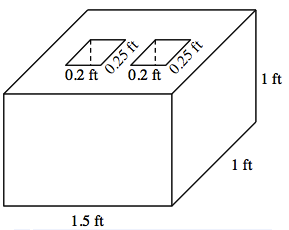### Home > INT2 > Chapter 12 > Lesson 12.2.2 > Problem12-93

12-93.

A cement block is the shape of a rectangular prism with length $1.5$ ft, width $1$ ft, and height $1$ ft.  Centered on the top of the block and passing all the way through the block are two $0.25$ ft by $0.2$ ft rectangular holes.

1. Draw a diagram of the block.

2. What is the volume of the block?Subtract the volumes of the two inner prisms from the volume of the large outer prism.